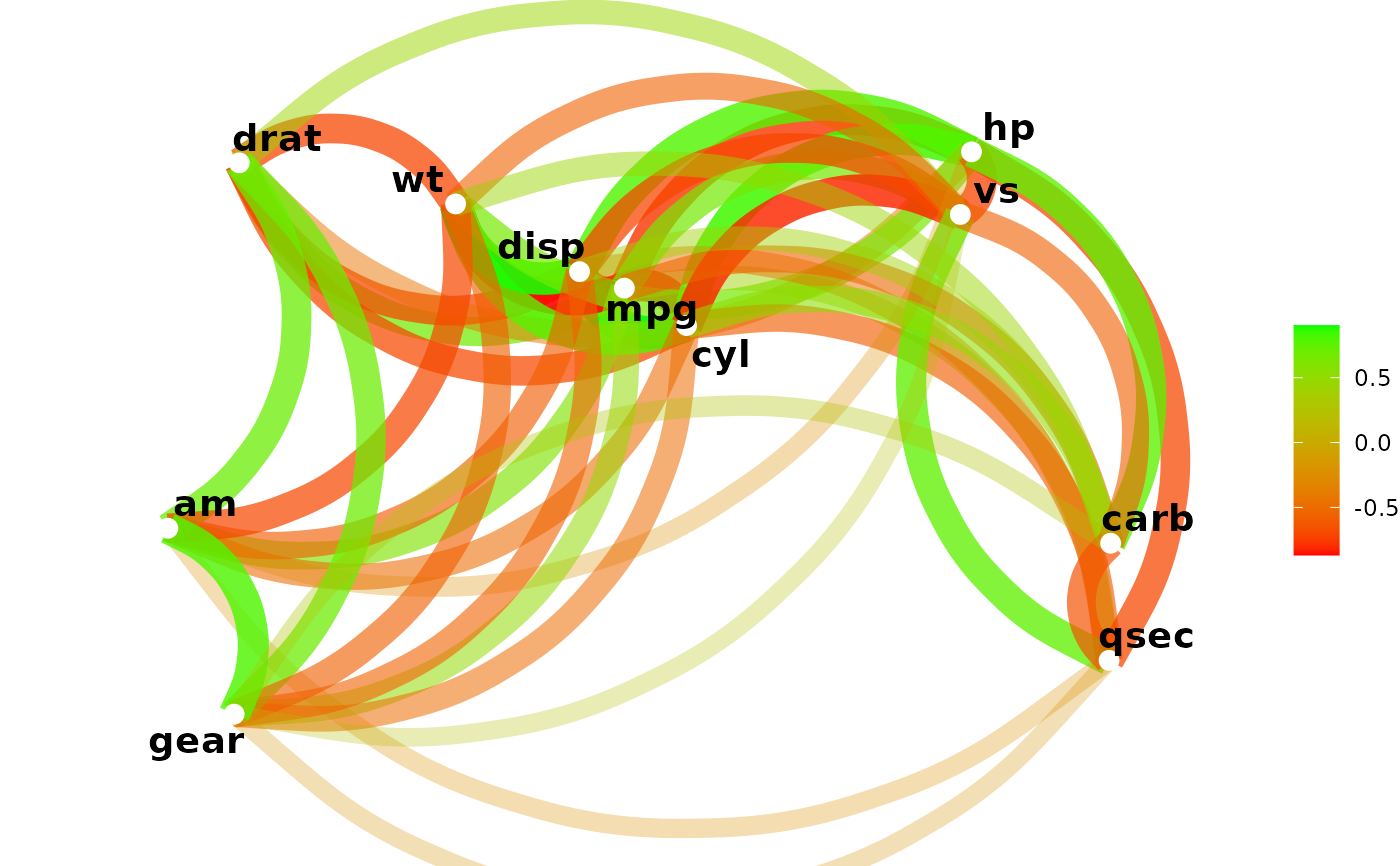Output a network plot of a correlation data frame in which variables that are more highly correlated appear closer together and are joined by stronger paths. Paths are also colored by their sign (blue for positive and red for negative). The proximity of the points are determined using multidimensional clustering.

## Usage

network_plot(
rdf,
min_cor = 0.3,
legend = c("full", "range", "none"),
colours = c("indianred2", "white", "skyblue1"),
repel = TRUE,
curved = TRUE,
colors
)

## Arguments

rdf

Correlation data frame (see correlate) or object that can be coerced to one (see as_cordf).

min_cor

Number from 0 to 1 indicating the minimum value of correlations (in absolute terms) to plot.

legend

How should the colors and legend for the correlation values be displayed? The options are "full" (the default) for -1 to 1 with a legend, "range" for the range of correlation values in rdf with a legend, or "none" for colors between -1 to 1 with no legend displayed.

colours, colors

Vector of colors to use for n-color gradient.

repel

Should variable labels repel each other? If TRUE, text is added via geom_text_repel instead of geom_text

curved

Should the paths be curved? If TRUE, paths are added via geom_curve; if FALSE, via geom_segment

## Examples

x <- correlate(mtcars)
#> Correlation computed with
#> • Method: 'pearson'
#> • Missing treated using: 'pairwise.complete.obs'
network_plot(x)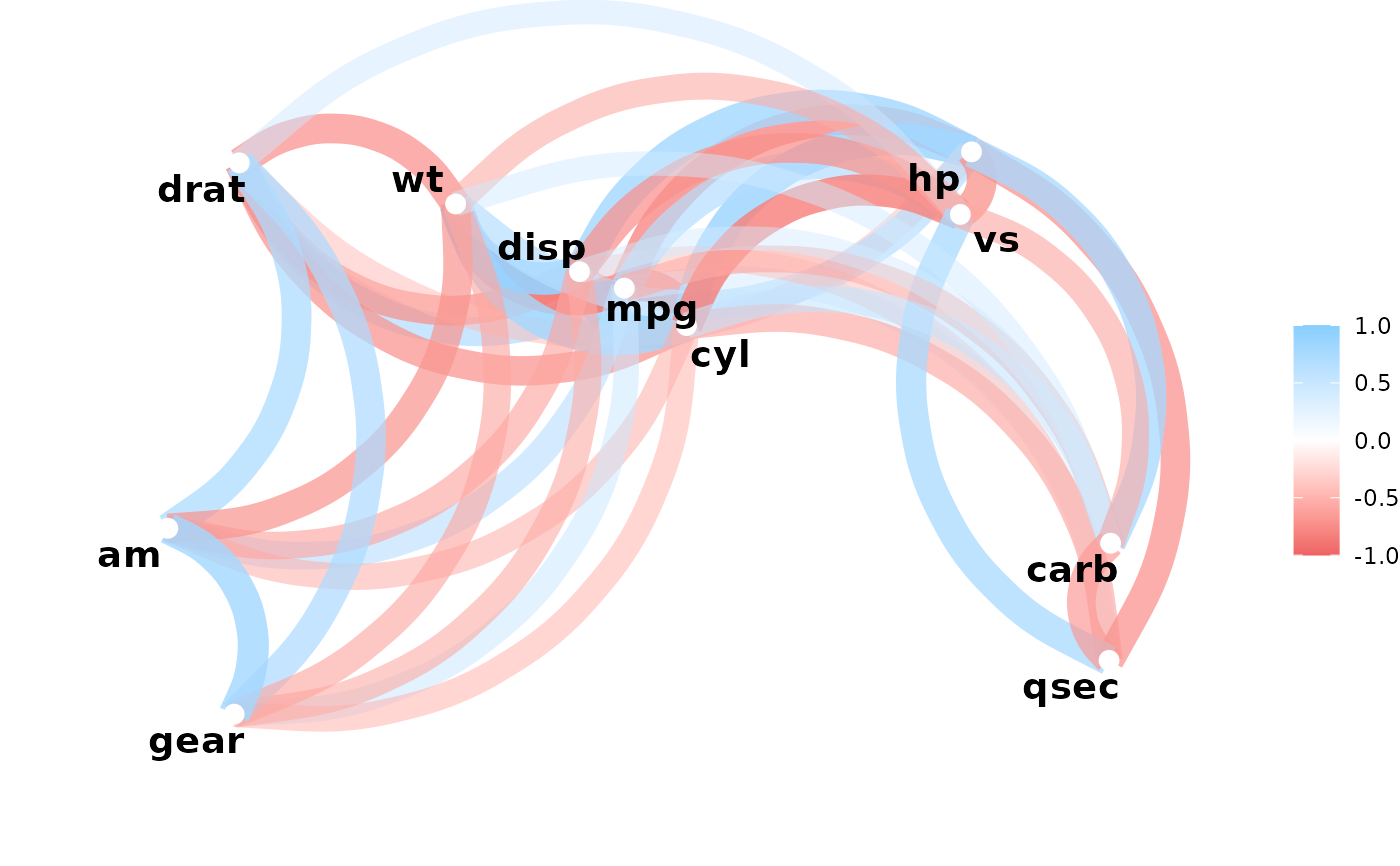network_plot(x, min_cor = .1)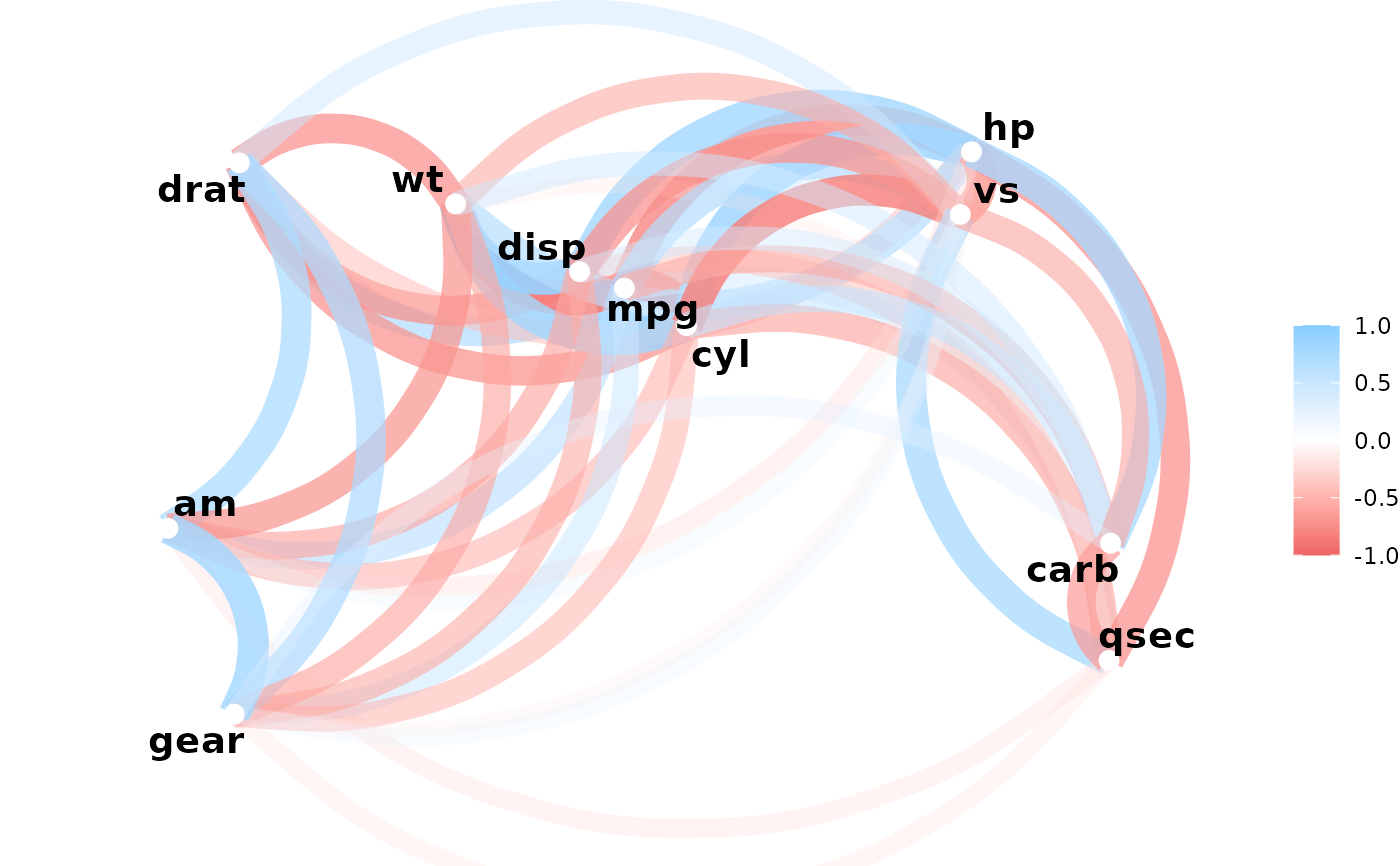network_plot(x, min_cor = .6)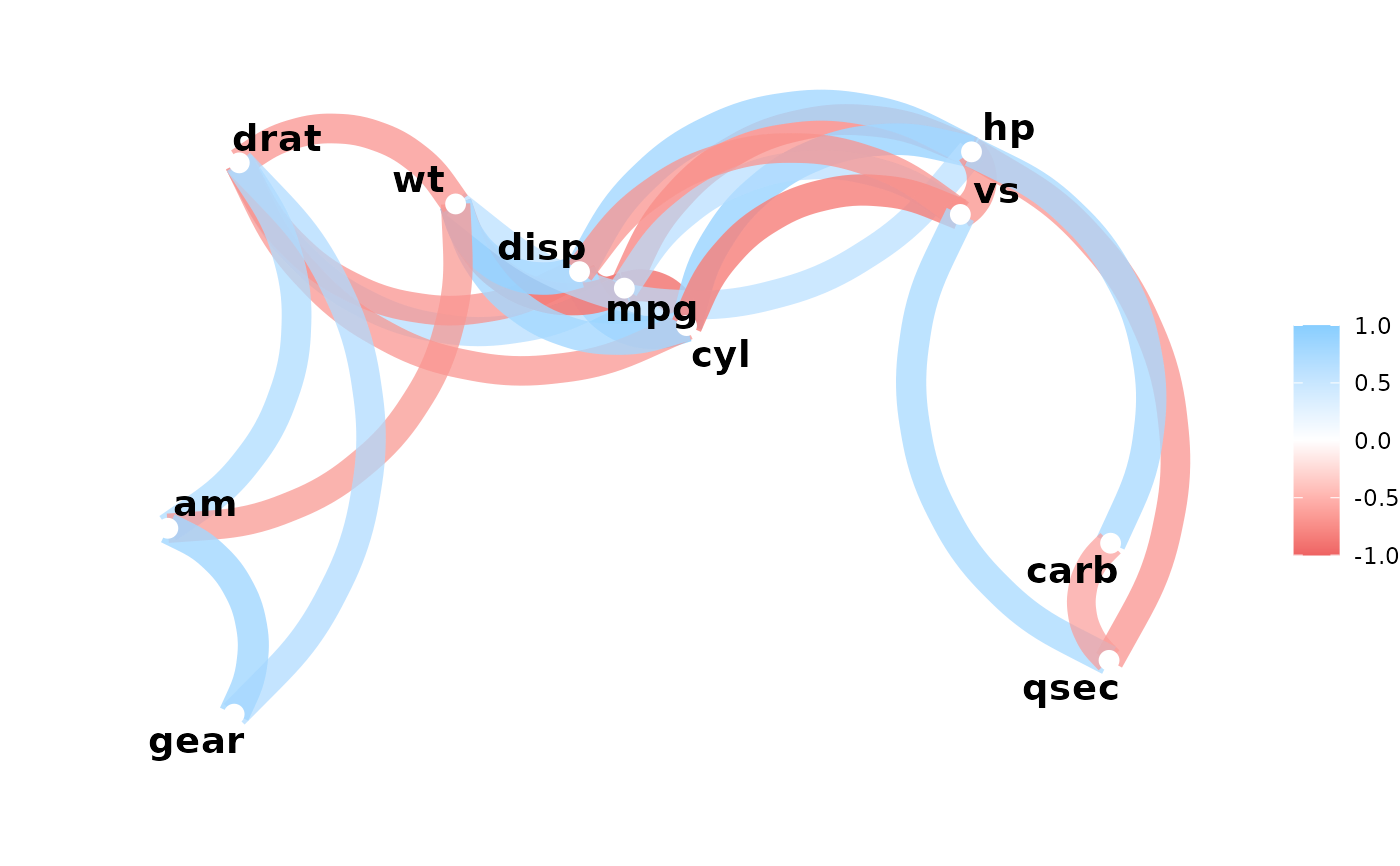network_plot(x, min_cor = .2, colors = c("red", "green"), legend = "full")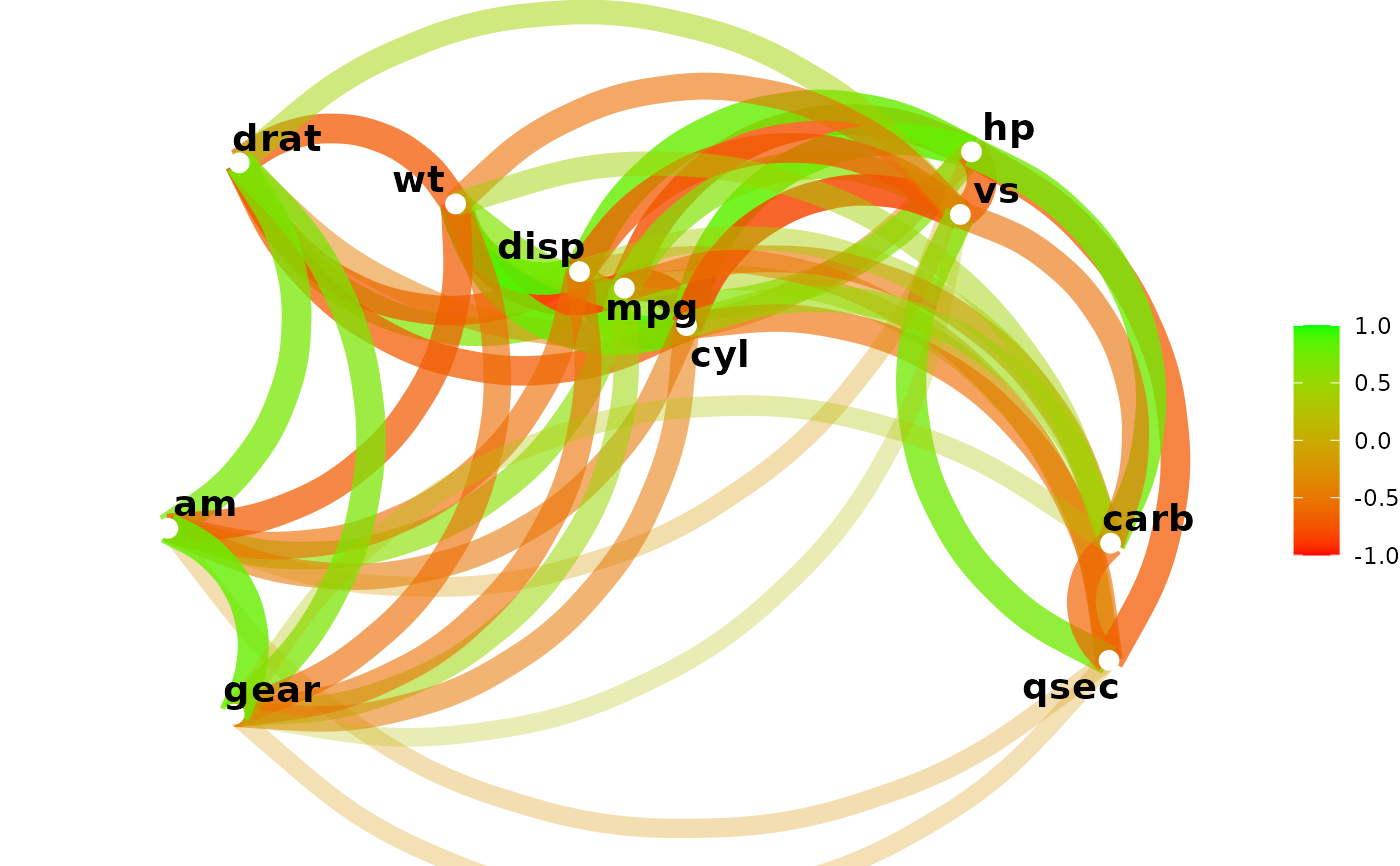network_plot(x, min_cor = .2, colors = c("red", "green"), legend = "range")